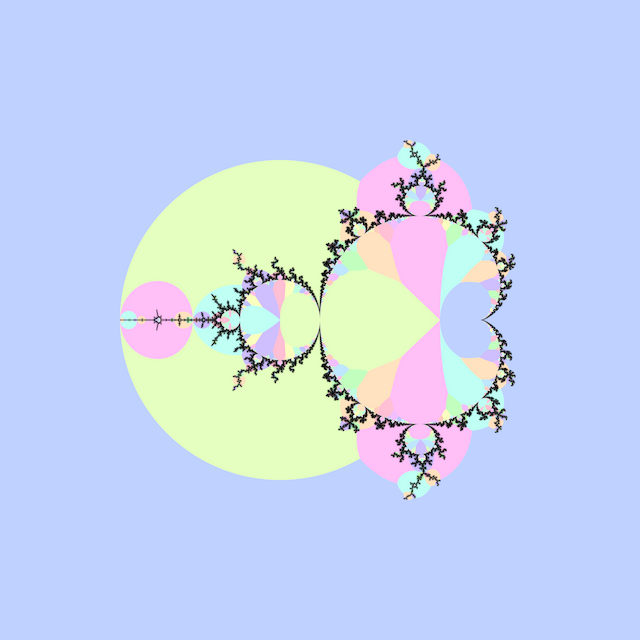# # Atom DomainsRobert P. Munafo “Atom Domain” (1996) and Heinz-Otto Peitgen and Peter H. Richter’s “The Beauty of Fractals: Images of Complex Dynamical Systems” (1986) [figure 34 on page 61] describe atom domains in the Mandelbrot set, defined as the index $$p \ge 1$$ at which $$\left|z_p\right|$$ is minimized during iteration of $$z_0 = 0$$ and $$z_{n+1} = z_n^2 + c$$. Atom domains surround hyperbolic components of the same period, and are generally much larger than the components themselves, which makes them useful for finding components.

Modified Atom Domains (2012) describes a modification of atom domains, which makes smaller domains more visible.

## # 1 C99 Code

#include <complex.h>
#include <math.h>

int m_atom_domains(int N, double R, double _Complex c)
{
double _Complex z = c;
double mp = 1.0 / 0.0;
int p = 0;
for (int n = 1; n < N; ++n)
{
double zp = cabs(z);
if (zp < mp)
{
mp = zp;
p = n;
}
z = z * z + c;
}
return p;
}